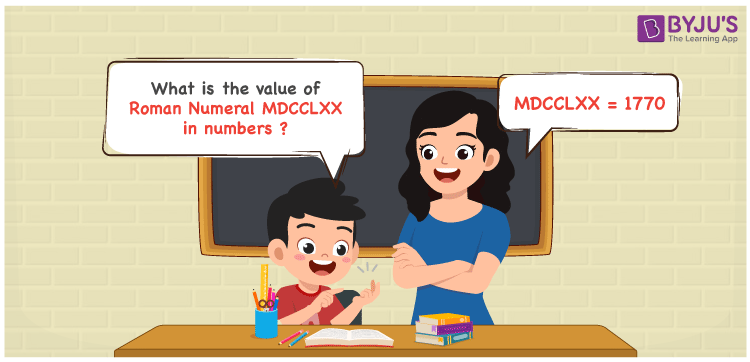Checkout JEE MAINS 2022 Question Paper Analysis : Checkout JEE MAINS 2022 Question Paper Analysis :

# MDCCLXX Roman Numerals

MDCCLXX Roman Numerals is 1770. In this article, MDCCLXX is expanded and written in numbers using the symbols chart. If you learn all the concepts in the roman numerals conversion article, you will be able to face the final exams effortlessly. Therefore, the roman numerals MDCCLXX is written as 1770 in numbers.

 Number Roman Numeral 1770 MDCCLXX## How to Write MDCCLXX Roman Numerals in Numbers?

In this section, MDCCLXX is converted to numbers in a stepwise manner.

MDCCLXX = M + D + C + C + L + X + X

MDCCLXX = 1000 + 500 + 100 + 100 + 50 + 10 + 10

MDCCLXX = 1770

## Video Lesson on Roman Numerals## Frequently Asked Questions on MDCCLXX Roman Numerals

### How is MDCCLXX the roman numerals of 1770?

We know that

M is the roman numerals of 1000

DCC is the roman numerals of 700

LXX is the roman numerals of 70

So MDCCLXX is the roman numeral of 1770.

### Determine 1800 – 30.

We know that

1800 – 30 = 1770

Hence, 1800 – 30 is 1770 which is written as MDCCLXX.

### Calculate the remainder we get MDCCLXX is divided by XIV.

We know that

MDCCLXX = 1770

XIV = 14

The remainder we get MDCCLXX is divided by XIV is VI.